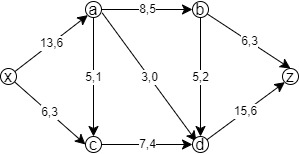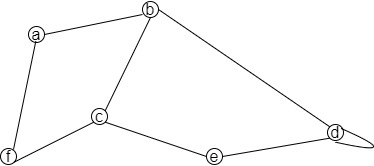### Exam Year

Tribhuvan University

Institute of Science and Technology

2080

Bachelor Level / second-semester / Science

Computer Science and Information Technology( CSC160 )

Discrete Structure

Full Marks: 60 + 20 + 20

Pass Marks: 24 + 8 + 8

Time: 3 Hours

Candidates are required to give their answers in their own words as far as practicable.

The figures in the margin indicate full marks.

Section A

Attempt any two questions.

1

Explain strong induction in detail. What is recursively defined function? Use mathematical induction to prove 7n+2 + 82n+1  is divisible by 5

2

State pigeonhole principle. Solve the recurrence relation an = 5an-1 – 6an-2 with initial conditions a0 = 1 and a1 = 3.

3

State max flow min cut theorem. Find the value of maximal flow in the graph below:Section B

Attempt any eight questions.

4

Explain the principle of inclusion and exclusion. How many integers from 1 to 30 are multiples of 2 or 3?

5

Give the example of ceiling, floor and boolean function. How do you plot the graph of the function?

6

Using Chinese remainder theorem solve the following congruences.

x = 1 (MOD 3)

x = 3 (MOD 5)

x = 6 (MOD 7)

7

Find the multiplicative inverse of 4 in Z11 using extended euclidean algorithm.

8

Express the following sentences using quantifier.

a. Not all people are loyal.

b. Everybody loves somebody.

c. Someone has passed the exam.

d. Aquatic animals can’t live without water.

e. Some subjects are not interesting.

9

What is proof by contradiction? Give a proof by contradiction to show that if 3n+2 is odd then n is odd.

10

State the necessary conditions for two graphs to be isomorphic. How many different words from “MANAGER” can be generated with or without meaning?

11

Define symmetric closure. What is the symmetric closure of the relation R = {(1,1), (1,2), (2,2), (2,3), (3,1), (3,2)} on the set A = {1,2,3}?

12

Represent following graph using adjacency matrix.